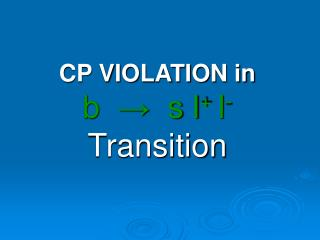DownloadDownload PresentationCP VIOLATION in b → s l + l - Transition

# CP VIOLATION in b → s l + l - Transition

Télécharger la présentation## CP VIOLATION in b → s l + l - Transition

- - - - - - - - - - - - - - - - - - - - - - - - - - - E N D - - - - - - - - - - - - - - - - - - - - - - - - - - -
##### Presentation Transcript

1. CP VIOLATION in b → s l+ l- Transition

2. Direct CP-Violation CP non-conservation shows up as a rate difference between two processes that are the CP conjugates of one-another. How can such a rate difference appear?

3. This shows that the effect will vanish if the two coupling constants can be made relatively real. And also if strong phase be zero.

4. CP violation in SM. 4 parameters : Euler angles: ½ n(n-1)=3 Complex Phase: ½ (n-1)(n-2)=1 Unitarity and Properties of CKM

5. physics beyond the Standard Model will have, in general, possible additional CP-violating parameters. Any further fields, such as any additional Higgs fields, can introduce further CP-violating couplings. Such effects may then enter into B decay physics.

6. FCNC IN b s(d) TRANSITION : 1 . As a test of SM and beyond SM 2. As a tool to Det. CKM elements such as Vtq(q=d,s,b), Vub 3. CP- Violation

7. b → s l+ l- Vub Vus* + Vtb Vts* + Vcb Vcs* = 0 Using unitary condition of CKM matrix and neglecting |VubV*us| in comparison to |VtbV*ts| and |VcbV*cs| Indicate that such decay involves only CP –Violation in this channel is suppressedby SM.

8. Beyond the SM • The possibility of CP violation the b → s transition has been studied in supersymmetry by [Kruger, et al]. • The SM with fourth generation [Arhrib et al]. • In another study, this has been studied with the addition of CP odd phases to Wilson coefficients [Aliev, et al].

9. The formalism • Using general form of 4-Fermi interactions in SM.

10. We extend the matrix element of the b → s l+ l- where C9get a new weak phase. Minimal Extension of the SM: ΛnewCan be parameterized as :

11. Using the expression of the matrix element and neglecting the s-quark mass(ms), we obtain the expression for the differential decay rate as:

12. is the velocity of the final lepton & ∆ is expression in terms of masses , Wilson coefficients.

13. CP-Violation asymmetry • CP-Violation differential decay width asymmetry is defined:

14. The differential decay width for the CP conjugated process can be obtain by making the replacements:

15. CP-asymmetry is evaluated to be Since for any allowed region of s,  << , So, we can ignore the term proportional to in the dominator of equation:

16. Numerical analysis • we examine the dependence of ACP on new .. • ACP depends on both magnitude and phase of new . • The first measurement of the b → s l+ l- decay reported by BELLE help us to get an idea about magnitude of new. We assume that:

17. In order to eliminate the sdependence, instead of CP asymmetry in the differential decay width, we study CP asymmetry in the total decay width by doing numerical integration over sin ACP(s) equation:

18. The figure depicts that ACP is sensitive to the new weak phase and can reach about 4.5% which is quite measurable at future colliders such as LHCb, BTeV, ATLAS CMS or ILC .

19. In conclusion, this study has presented the CP asymmetry in the b → s l+ l- transition in the minimal extension of the standard model where C9effreceived extra weak phase new due to the new physics effects. We imposed 10% of uncertainty to the SM branching ratio of the b → s l+ l- transition and obtained the bound on a new parameter new . Our predictive model showed that the CP-violation asymmetry could reach to the order of 4.5% which was not only entirely measurable in experiments, but also indicated the new physics effects, since in the SM, this CP asymmetry is near zero.